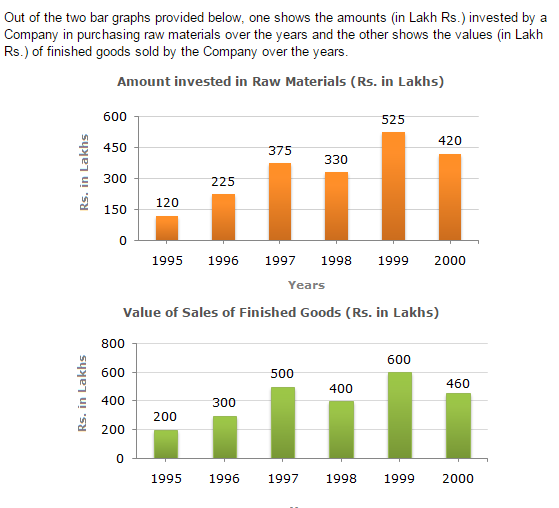Direction1.

The maximum difference between the amount invested in Raw materials and value of sales of finished goods was during the year?

 A. 1995 B. 1996 C. 1997 D. 1998
Explanation: The differences between the amount invested in raw material and the value of sales of finished goods for various years are: For 1995 = Rs. (200 - 120) lakhs = Rs. 80 lakhs. For 1996 = Rs. (300 - 225) lakhs = Rs. 75 lakhs. For 1997 = Rs. (500 - 375) lakhs = Rs. 125 lakhs. For 1998 = Rs. (400 - 330) lakhs = Rs. 70 lakhs. For 1999 = Rs. (600 - 525) lakhs = Rs. 75 lakhs. For 2000 = Rs. (460 - 420) lakhs = Rs. 40 lakhs. Clearly, maximum difference was during 1997.

2.

The value of sales of finished goods in 1999 was approximately what percent of the sum of amount invested in Raw materials in the years 1997, 1998 and 1999?

 A. 33% B. 37% C. 45% D. 49%
Explanation: Required percentage = [ 600 x 100 ] % (375 + 330 + 525) = 48.78% ~= 49%.

3.

What was the difference between the average amount invested in Raw materials during the given period and the average value of sales of finished goods during this period?

 A. Rs. 62.5 lakhs B. Rs. 68.5 lakhs C. Rs. 71.5 lakhs D. Rs. 77.5 lakhs
Explanation: Required difference = Rs. [ 1 x (200 + 300 + 500 + 400 + 600 +460) 6 - 1 x (120 + 225 + 375 + 330 + 525 + 420) ] lakhs 6 = Rs. [ ( 2460 ) - ( 1995 ) ] lakhs 6 6 = Rs. (410 - 332.5) lakhs = Rs. 77.5 lakhs.

4.

In which year, the percentage change (compared to the previous year) in the investment on Raw materials is same as that in the value of sales of finished goods?

 A. 1996 B. 1997 C. 1998 D. 1999